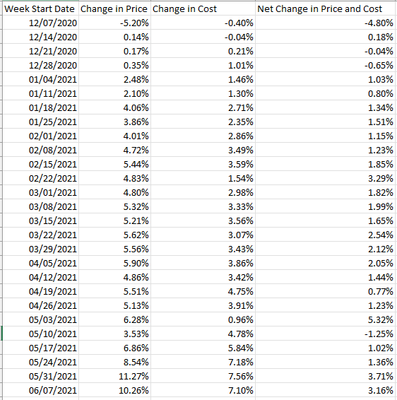cancel
Showing results for
Did you mean:Helper III

## DAX in building Rolling 4 Week Average

Hi,

I need help in building 4 week rolling average for "Net Change in Price and Cost" with below sample dataChange in Price, Change in Cost and Net Change in Price and Cost are all 3 measures.

Net Change in Price and Cost = Change in Price - Change in Cost

I do have separate date table and the above data shows weekly data and my report has Invoice Date as Filter.

I need to calculate rolling 4 week average, here the tweak is that for first week start date 12/07 rolling average should be same while for next 12/14 it should (-4.80% + 0.18%)/2 = -2.31%

for 12/21 it should be (-4.80% + 0.18% - 0.04%)/3 = -1.55%

for 12/28 it should be (-4.80% + 0.18% - 0.04% -0.65%)/4 = -1.33%

for 01/04 it should be (0.18% - 0.04% -0.65% +1.03%)/4 = 0.13%

as shown belowI have created below DAX but its not working as expected

Net Change in Price and Cost 4 Wk Rolling Avg =
var start_day = MIN('Date'[Week Start Date])-21
var end_day = MAX('Date'[Week Start Date])

return
CALCULATE([Net Change in Price and Cost],
DATESBETWEEN('Date'[Week Start Date],start_day,end_day),
REMOVEFILTERS('Date'[Week Start Date]))/CALCULATE(DISTINCTCOUNT('Date'[Week Start Date]),DATESBETWEEN('Date'[Week Start Date],start_day,end_day),REMOVEFILTERS('Date'[Week Start Date]))

Can someone help me on this??

1 ACCEPTED SOLUTIONCommunity Support

Hi @apatwal ,

Here's my solution.

1.Create a index measure

``````Index =
CALCULATE (
COUNT ( 'Date'[Week Start Date] ),
FILTER (
ALL ( 'Date' ),
[Week Start Date] <= MAX ( 'Table'[Week Start Date] )
)
)
``````2.Create another measure to get the rolling 4 week average.

``````Rolling 4 week average =
CALCULATE (
AVERAGEX ( 'Table', [Net Change in Prce and Cost] ),
FILTER (
ALL ( 'Table' ),
[Index] <= MAXX ( 'Table', [Index] )
&& [Index]
>= MAXX ( 'Table', [Index] ) - 3
)
)
``````Best Regards,

Stephen Tao

If this post helps, then please consider Accept it as the solution to help the other members find it more quickly.

4 REPLIES 4Community Support

Hi @apatwal ,

Here's my solution.

1.Create a index measure

``````Index =
CALCULATE (
COUNT ( 'Date'[Week Start Date] ),
FILTER (
ALL ( 'Date' ),
[Week Start Date] <= MAX ( 'Table'[Week Start Date] )
)
)
``````2.Create another measure to get the rolling 4 week average.

``````Rolling 4 week average =
CALCULATE (
AVERAGEX ( 'Table', [Net Change in Prce and Cost] ),
FILTER (
ALL ( 'Table' ),
[Index] <= MAXX ( 'Table', [Index] )
&& [Index]
>= MAXX ( 'Table', [Index] ) - 3
)
)
``````Best Regards,

Stephen Tao

If this post helps, then please consider Accept it as the solution to help the other members find it more quickly.Anonymous
Not applicable

Thanks so much. This really worked for me. Hope to learn a lot from these communitySuper User

@apatwal , Create a week rank on week start date or on week year

new columns

Week Rank = RANKX(all('Date'),'Date'[Week Start date],,ASC,Dense)
OR
Week Rank = RANKX(all('Date'),'Date'[Year Week],,ASC,Dense) //YYYYWW format

Avg of rolling 4 weeks , assuming [Net Change in Price and Cost] is measure

Last 4 weeks = CALCULATE(Averagex(Values('Date'[Week Rank]), [Net Change in Price and Cost]), FILTER(ALL('Date'),'Date'[Week Rank]>=max('Date'[Week Rank])-4 && 'Date'[Week Rank]<=max('Date'[Week Rank])))Helper III

But looks there is some issue in calculationsUsed same DAX provided by you..

Rolling 4 Week Average =
CALCULATE(
AVERAGEX(
VALUES('Date'[Week Rank]),
MainTable[Net Change in Price and Cost]
),
FILTER(
ALL('Date'),
'Date'[Week Rank] >= MAX('Date'[Week Rank])-4 &&
'Date'[Week Rank] <= MAX('Date[Week Rank])
)
)